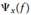Create an Account

Home / Questions / 1 Consider two energy signals g1t and g2t These two signals are delayed by amounts equal t...

1 Consider two energy signals g1t and g2t These two signals are delayed by amounts equal to t1 and t2 seconds respectively Show that the time delays are additive in convolving the pair of delay

1. Consider two energy signals g1(t) and g2(t) These two signals are delayed by amounts equal to tand t2 seconds, respectively. Show that the time delays are additive in convolving the pair of delayed signals, whereas they are subtractive in cross-correlating them.

2. (a) An energy signal  x(t), its Fourier transform X(f), autocorrelation function Rx (τ), and energy spectral density are all related, directlyor indirectly. Construct a flow-graph that portrays all the possible direct relationships between them.

(b) If you are given the frequency-domain description X(f), the autocorrelation function Rx (τ), can be calculated from X(f) .Outline two ways in which this calculation can be performed.

Jul 31 2020 View more View LessSubscribe To Get Solution